# MicroFocus Reasoning Question

DIRECTIONS for the questions 1 to 5 : Study the given information carefully to answer the given question:

Eight people- E, F, G, H, Q, R, S and T are sitting around a square table (but not necessarily in the same order) in such a way that four of them sit at four corners while four sit in the middle of each of the four sides. The ones sitting at the corners are facing the centre and the ones sitting in the middle of the sides are facing outside (i.e. opposite to the centre).

Q sits in the middle of one of the sides. Only two people sit between Q and R. Only one person sits between R and E. E is an immediate neighbor of both T and F. S sits to the immediate left of F. Only one person sits between G and S.

A. only conclusion II is true
B. either conclusion I or II is true
C. both only conclusion I is true
D. conclusions I and II are true
E. Neither conclusion I nor II is true
1. Who sits third to the left of G?
1. H
2. T
3. F
4. R
5. E

From the given information following arrangement can be drawn:F sits third to the left of G.
2. What is the position of T with respect to R?
1. Second to the right
2. Third to the left
3. Immediate left
4. Third to the right
5. Immediate right

From the given information following arrangement can be drawn:T is sitting to the immediate right of R.
3. How many people sit between T and F when counted from the left of T?
1. One
2. None
3. Three
4. Two
5. More than three

From the given information following arrangement can be drawn: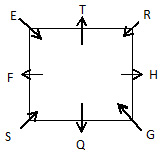Only one person sit between T and F when counted from the left of T.
4. Which of the following is true with respect to the given arrangement?
1. Q is an immediate neighbor of S.
2. F sits second to the left of H
3. H sits at one of the corners of the table
4. None of the given statements is true

From the given information following arrangement can be drawn: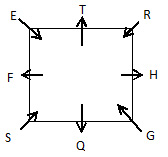Q is an immediate neighbor of S’ is the only true statement out of the given statements.
5. Which of the following pairs represent the people sitting between Q and the one sitting second to the left of E, when counted from the left of Q?
1. F, S
2. H, G
3. F, G
4. T, S
5. R, T

From the given information following arrangement can be drawn: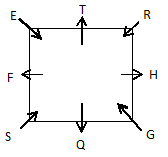The pair of persons sitting between Q and the one sitting second to the left of E (i.e., R) is H, G.
DIRECTIONS for questions 6-8 : In this question,two/three statements followed by two conclusions are given. You have to take the given statements to be true even if they seems to be at variance from commonly known facts and then decide which of the given conclusions logically follows disregarding commonly known facts. Mark your answer as:
6. Statements:All papers are woods. Some woods are leaves. All leaves are trunks.
Conclusion I:Some leaves are papers.
Conclusion II:At least some trunks are woods.

From the diagram, it can be obsereved that only conclusion II follows follow from the given statements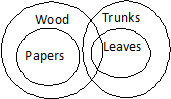7. Statements:No mobile is a band. All bands are pillows. Some pillows are sheets.
Conclusion I:No mobile is a pillow.
Conclusion II:All sheets are bands.

From the diagram, obsereve that no conclusion follows from the8. Statements: All papers are woods. Some woods are leaves. All leaves are trunks.
Conclusion I:All trunks being woods is a possibility.
Conclusion II:Some trunks are papers.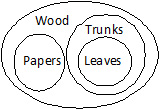From the diagram, it can be observed that only conclusion I follows from the given statements
9. Among five people- A, B, C, D and E, each scoring different marks, only two persons scored more marks than A. D scored more than A. B scored less than D but not the lowest. C scored more than B but not the highest. Who scored the lowest marks?
1. Cannot be determined
2. B
3. E
4. C
5. D

According to the given information, the five people can be arranged in the
decreasing order of their marks as follows:
D > C > A > B > E.
Thus, E scored the lowest marks.
10. Four of the following five are alike in a certain way (based on their positions of alphabets in
the English alphabetical series) and hence form a group. Which is the one that does not belong to that group?
1. BFD
2. EIH
3. KOM
4. TXV
5. LPN